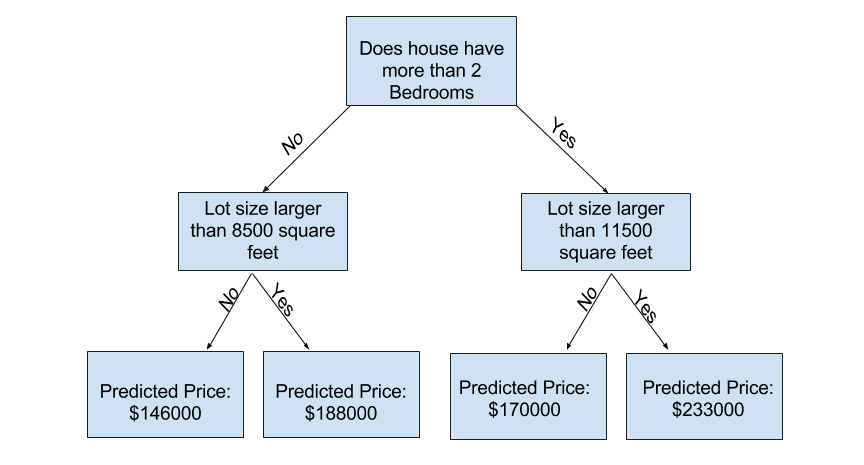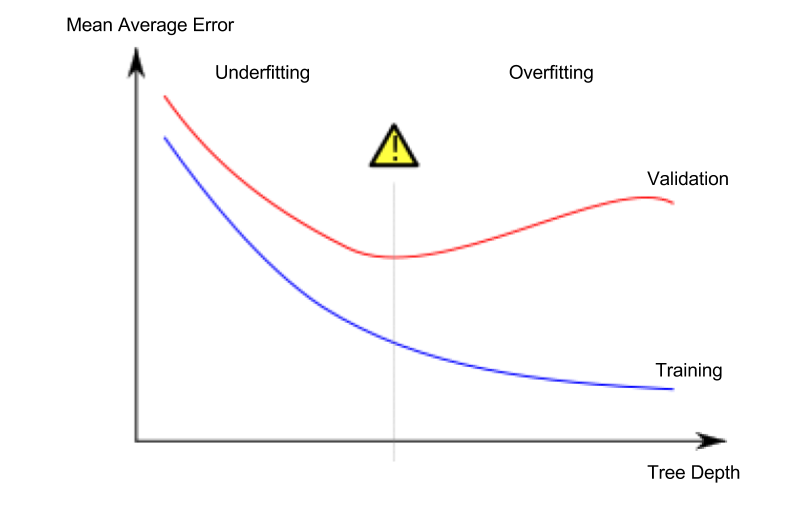# Kaggle Challenge 10 - Underfitting and Overfitting

ljsk99499·2021년 8월 12일
0

## Kaggle Challenge August 2nd~

목록 보기
8/12### @Tutorial### @Example

There are a few alternatives for controlling the tree depth, and many allow for some routes through the tree to have greater depth than other routes. But the max_leaf_nodes argument provides a very sensible way to control overfitting vs underfitting. The more leaves we allow the model to make, the more we move from the underfitting area in the above graph to the overfitting area.
We can use a utility function to help compare MAE scores from different values for max_leaf_nodes:

from sklearn.metrics import mean_absolute_error
from sklearn.tree import DecisionTreeRegressor

def get_mae(max_leaf_nodes, train_X, val_X, train_y, val_y):
model = DecisionTreeRegressor(max_leaf_nodes=max_leaf_nodes, random_state=0)
model.fit(train_X, train_y)
preds_val = model.predict(val_X)
mae = mean_absolute_error(val_y, preds_val)
return(mae)
# compare MAE with differing values of max_leaf_nodes
for max_leaf_nodes in [5, 50, 500, 5000]:
my_mae = get_mae(max_leaf_nodes, train_X, val_X, train_y, val_y)
print("Max leaf nodes: %d  \t\t Mean Absolute Error:  %d" %(max_leaf_nodes, my_mae))
Max leaf nodes: 5  		 Mean Absolute Error:  347380
Max leaf nodes: 50  		 Mean Absolute Error:  258171
Max leaf nodes: 500  		 Mean Absolute Error:  243495
Max leaf nodes: 5000  		 Mean Absolute Error:  254983

### Step 1: Exercises

Quesition

You could write the function get_mae yourself. For now, we'll supply it. This is the same function you read about in the previous lesson. Just run the cell below.

def get_mae(max_leaf_nodes, train_X, val_X, train_y, val_y):
model = DecisionTreeRegressor(max_leaf_nodes=max_leaf_nodes, random_state=0)
model.fit(train_X, train_y)
preds_val = model.predict(val_X)
mae = mean_absolute_error(val_y, preds_val)
return(mae)
candidate_max_leaf_nodes = [5, 25, 50, 100, 250, 500]
# Write loop to find the ideal tree size from candidate_max_leaf_nodes
_

# Store the best value of max_leaf_nodes (it will be either 5, 25, 50, 100, 250 or 500)
best_tree_size = ____

step_1.check()

Solution

# Here is a short solution with a dict comprehension.
# The lesson gives an example of how to do this with an explicit loop.
scores = {leaf_size: get_mae(leaf_size, train_X, val_X, train_y, val_y) for leaf_size in candidate_max_leaf_nodes}
best_tree_size = min(scores, key=scores.get)

### Step 1: Fit Model Using All Data

Quesition

You know the best tree size. If you were going to deploy this model in practice, you would make it even more accurate by using all of the data and keeping that tree size. That is, you don't need to hold out the validation data now that you've made all your modeling decisions.

# Fill in argument to make optimal size and uncomment
# final_model = DecisionTreeRegressor(____)

# fit the final model and uncomment the next two lines
# final_model.fit(____, ____)

step_2.check()

Solution

# Fit the model with best_tree_size. Fill in argument to make optimal size
final_model = DecisionTreeRegressor(max_leaf_nodes=best_tree_size, random_state=1)

# fit the final model
final_model.fit(X, y)

### @Example02

• train_X
• val_X
• train_y
• val_y
We build a random forest model similarly to how we built a decision tree in scikit-learn - this time using the RandomForestRegressor class instead of DecisionTreeRegressor.
from sklearn.ensemble import RandomForestRegressor
from sklearn.metrics import mean_absolute_error

forest_model = RandomForestRegressor(random_state=1)
forest_model.fit(train_X, train_y)
melb_preds = forest_model.predict(val_X)
print(mean_absolute_error(val_y, melb_preds))
191669.7536453626

### Step 1: Use a Random Forest

Quesition

Data science isn't always this easy. But replacing the decision tree with a Random Forest is going to be an easy win.

from sklearn.ensemble import RandomForestRegressor

# Define the model. Set random_state to 1
rf_model = ____

____

# Calculate the mean absolute error of your Random Forest model on the validation data
rf_val_mae = ____

print("Validation MAE for Random Forest Model: {}".format(rf_val_mae))

step_1.check()

Solution

rf_model = RandomForestRegressor()

rf_val_mae = mean_absolute_error(rf_val_predictions, val_y)